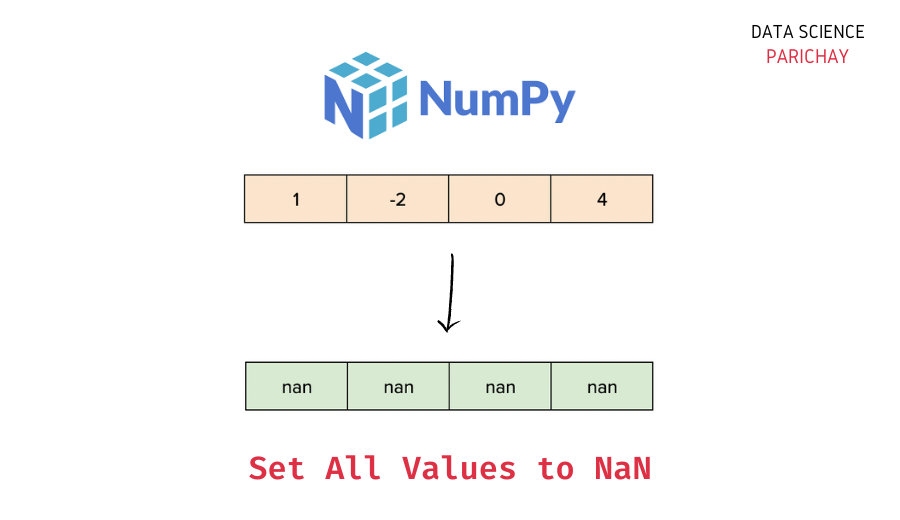# Numpy – Set All Values to Nan in Array

The Numpy library in Python comes with a number of built-in functions to manipulate the data in arrays. In this tutorial, we will look at a function that helps us set all the values in a Numpy array to nan.

## How to set all values to nan in Numpy?

You can use the `numpy.ndarray.fill()` function to set all the values in a Numpy array to nan. Pass `numpy.nan` as the argument (this is the value used to fill all the values in the array).

The following is the syntax –

```# set all values in numpy array ar to nan
ar.fill(np.nan)```

It modifies the array in-place, filling each value with nan (the passed value).

Let’s now look at a step-by-step example of using the above function.

### Step 1 – Create a Numpy array

First, we will create a Numpy array that we will use throughout this tutorial.

```import numpy as np

# create a numpy array
ar = np.array([-2, -1, 0, 1, 2, 3, -4.1])
# display the array
print(ar)```

Output:

`[-2.  -1.   0.   1.   2.   3.  -4.1]`

Here, we used the `numpy.array()` function to create a Numpy array containing some numbers.

📚 Data Science Programs By Skill Level

Introductory

Intermediate ⭐⭐⭐

🔎 Find Data Science Programs 👨‍💻 111,889 already enrolled

Disclaimer: Data Science Parichay is reader supported. When you purchase a course through a link on this site, we may earn a small commission at no additional cost to you. Earned commissions help support this website and its team of writers.

### Step 2 – Set each value to nan using `numpy.ndarray.fill()`

Apply the `numpy.ndarray.fill()` function on the array and pass `numpy.nan` as the parameter to set each value to nan in the array.

Let’s apply this function to the array created above.

```# set all values to nan
ar.fill(np.nan)
# display the array
ar```

Output:

`array([nan, nan, nan, nan, nan, nan, nan])`

You can see that each value in the array `ar` is now nan.

Note that if all the values in the array are of integer type, using the above method will fill the array with zeros instead of nans.

```# create a numpy array
ar = np.array([-2, -1, 0, 1, 2, 3, -4])
# set all values to nan
ar.fill(np.nan)
# display the array
ar```

Output:

`array([0, 0, 0, 0, 0, 0, 0])`

This happens because `np.nan` is of `float` type which is being used to fill an integer array.

In order to prevent this behavior and fill the array with nans, first convert the array to `float` type and then apply the `numpy.ndarray.fill()` function.

```# create a numpy array
ar = np.array([-2, -1, 0, 1, 2, 3, -4])
# convert to float
ar = ar.astype('float')
# set all values to nan
ar.fill(np.nan)
# display the array
ar```

Output:

`array([nan, nan, nan, nan, nan, nan, nan])`

Now all the values in the array are nan.

You might also be interested in –

•